# 平面图的变换与着色的性质Moves and Coloring Properties for Planar Graph

DOI: 10.12677/AAM.2021.105160, PDF, HTML, XML, 下载: 41  浏览: 92  国家自然科学基金支持

Abstract: This paper studies the coloring properties of planar graph, gives their moves including tree-move triangle-move (n-circle-move), bridge-move, and proves that these moves don’t change coloring number by using dichromatic polynomial. Furthermore, the coloration of some planar graphs is studied.

1. 引言

2. 预备知识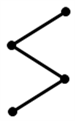Figure 1. 3-arc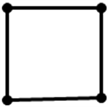Figure 2. 4-loop

(1) $Z\left({•}_{}^{}G\right)=q$

(2) $Z\left({•}_{}^{}G\right)=qZ\left( G \right)$

(3) $Z$ (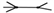) $=Z$ (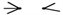) $\text{ }+vZ$ ()

$qP\left(G\right)=P\left(H\right)P\left(K\right)$

3. 平面图的变换及其着色性质

3.1. 树形变换的着色问题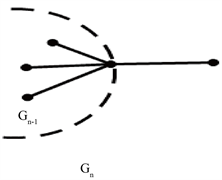Figure 3. 1-arc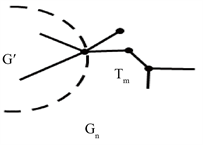Figure 4. Tree

3.2. “m–圈–变换”的着色问题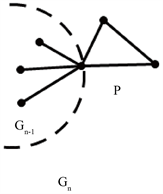Figure 5. Graph with 3-loop

(1) 当m是偶数时，如果 $q\ge 2$，则 $P\left({G}_{n}\right)\ne 0$ 当且仅当 $P\left({G}^{\prime }\right)\ne 0$

(2) 当m是奇数时，如果 $q\ge 3$，则 $P\left({G}_{n}\right)\ne 0$ 当且仅当 $P\left({G}^{\prime }\right)\ne 0$

$\begin{array}{c}Z\left({G}_{n}\right)=Z\left({G}^{\prime }\cup {T}_{m}\right)+vZ\left({G}^{\prime }\cup {P}_{m-1}\right)\\ =\left[{\left(q+v\right)}^{m-1}+v{\left(q+v\right)}^{m-2}+\cdots +{v}^{m-3}{\left(q+v\right)}^{2}\right]Z\left({G}^{\prime }\right)+{v}^{m-2}Z\left({G}^{\prime }\cup {P}_{1}\right)\\ =\left[{\left(q+v\right)}^{m-1}+v{\left(q+v\right)}^{m-2}+\cdots +{v}^{m-2}\left(q+v\right)\right]Z\left({G}^{\prime }\right)+{v}^{m-1}Z\left({G}^{\prime }\cup {P}_{1}\right)\\ =\left[{\left(q+v\right)}^{m-1}+v{\left(q+v\right)}^{m-2}+\cdots +{v}^{m-2}\left(q+v\right)+{v}^{m-1}\left(v+1\right)\right]Z\left({G}^{\prime }\right)\\ =\left[\frac{{\left(q+v\right)}^{m-1}\left[1-{\left(\frac{v}{q+v}\right)}^{m-1}\right]}{1-\frac{v}{q+v}}+{v}^{m-1}\left(v+1\right)\right]Z\left({G}^{\prime }\right)\\ =\left[\frac{\left(q+v\right)\left[{\left(q+v\right)}^{m-1}-{v}^{m-1}\right]}{q}+{v}^{m-1}\left(v+1\right)\right]Z\left( G \prime \right)\end{array}$

$v=-1$ 时， $P\left({G}_{n}\right)=\frac{q-1}{q}\left[{\left(q-1\right)}^{m-1}-{\left(-1\right)}^{m-1}\right]P\left({G}^{\prime }\right)$，所以，当m是偶数时，

$P\left({G}_{n}\right)=\left(q-1\right)\left[{\left(q-1\right)}^{m-1}+1\right]P\left({G}^{\prime }\right)$，此时，如果 $q\ge 2$，则 $P\left({G}_{n}\right)\ne 0$ 当且仅当 $P\left({G}^{\prime }\right)\ne 0$。当m是奇数时，

$P\left({G}_{n}\right)=\left(q-1\right)\left[{\left(q-1\right)}^{m-1}-1\right]P\left({G}^{\prime }\right)$。此时，如果 $q\ge 3$，则 $P\left({G}_{n}\right)\ne 0$ 当且仅当 $P\left({G}^{\prime }\right)\ne 0$。从而定理的结论成立。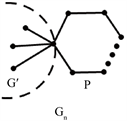Figure 6. Graph with m-loop

(1) 当m是偶数时，如果 $q\ge 2$，则 $P\left({G}_{n}\right)\ne 0$

(2) 当m是奇数时，如果 $q\ge 3$，则 $P\left({G}_{n}\right)\ne 0$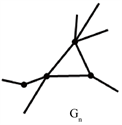Figure 7. Graph of 3-loop

3.3. 桥变换的着色问题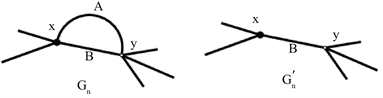Figure 8. Graph of multiple edges

(1) 当m为奇数时，如果 $q\ge 3$，则 $P\left({G}_{n}\right)\ne 0$ 当且仅当 $P\left({G}^{\prime }\right)\ne 0$

(2) 当m为偶数时，如果 $q\ge 2$，则 $P\left({G}_{n}\right)\ne 0$ 当且仅当 $P\left({G}^{\prime }\right)\ne 0$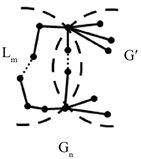Figure 9. Graph with bridge

$=\left[{\left(q+v\right)}^{m}+v{\left(q+v\right)}^{m-1}+{v}^{2}{\left(q+v\right)}^{m-2}+\cdots +{v}^{m-1}\left(q+v\right)\right]Z\left({G}^{\prime }\right)+\left(1+v\right)Z\left( G ″ \right)$

$v=-1$ 时， $P\left({G}_{n}\right)=\left[{\left(q-1\right)}^{m}-{\left(q-1\right)}^{m-1}+{\left(q-1\right)}^{m-2}+\cdots +{\left(-1\right)}^{m-1}\left(q-1\right)\right]P\left( G \prime \right)$

  Alexander, J.W. (1928) Topological Invariants of Knots and Links. Transactions of the American Mathematical Society, 30, 275-306. https://doi.org/10.1090/S0002-9947-1928-1501429-1  Conway, J.H. (1970) An Enumeration of Knots and Links, and Some of Their Algebraic Properties. Computational Problems in Abstract Algebra, New York, 29 August-2 September 1970, 329-358. https://doi.org/10.1016/B978-0-08-012975-4.50034-5  Jones, V.F.R. (1989) On Knot Invariants Related to Some Statistical Mechanical Models. Pacific Journal of Mathematics, 137, 311-334. https://doi.org/10.2140/pjm.1989.137.311  Kauffman, L.H. (1988) New Invariants in the Theory of Knots. The American Mathematical Monthly, 95, 195-242. https://doi.org/10.1080/00029890.1988.11971990  Wu, F.Y. (1992) Knot Theory and Statistical Mechanics. Modern Physics, 64, 1099-1131. https://doi.org/10.1103/RevModPhys.64.1099  Sumners, D.W. (1990) Untangling DNA. The Mathematical Intelligencer, 12, 71-80. https://doi.org/10.1007/BF03024022  Adams, C.C. (2004) The Knot Book. W. H. Freeman and Company, New York.  韩友发, 亢云凤, 董婷. 平面图的多项式与着色[J]. 辽宁师范大学学报, 2017, 40(3): 289-292.